#Function Repository Resource:

# HardSphereSimulation

Simulate hard spheres moving in an n-dimensional box

Contributed by: Matt Kafker and Christopher Wolfram
 ResourceFunction["HardSphereSimulation"][assoc] generates a simulation of the motion of a system of hard spheres with simulation parameters specified by assoc.

## Details

Input assoc must include the following:
 "Positions" initial particle positions "Velocities" initial particle velocities "BoxSize" side-length of simulation box "Steps" number of simulation time steps "StepSize" duration of a single time step "BoundaryCondition" interaction between particles and container walls "Output" simulation output
Input assoc may also include:
The dimensions of vectors in "Positions" and "Velocities" determine the spatial dimension of the system (they can be any positive integer dimension).
"ParticleMass" can either be a single number for identical-mass spheres or a list for spheres of different masses.
The box is centered on the origin.
Possible named boundary conditions include:
 "Reflecting" particle velocities reflect upon contact with container walls "Periodic" particles are translated to opposite side of box upon reaching container walls "Infinite" particles do not alter motion upon reaching container walls
Possible named outputs include:
 "PositionsByTime" list of all particle positions at every time step "VelocitiesByTime" list of all particle velocities at every time step "SpeedsByTime" list of all particle speeds at every time step "TrajectoriesByTime" list of all particle positions and velocities at every time step "PositionsByParticle" list of positions over time for each particle "VelocitiesByParticle" list of velocities over time for each particle "SpeedsByParticle" list of speeds over time for each particle "TrajectoriesByParticle" list of positions and velocities over time for each particle "Collisions" list of particle-particle collisions "All" list containing “TrajectoriesByTime” output as the first entry, and “Collisions” as the second "Visualize" Graphics object with a Manipulate slider for visualizing the time-evolution of the system in one, two, or three spatial dimensions "KineticEnergy" list of total kinetic energy at every time step "Temperature" list of temperature at every time step "CausalGraph" Graph object with nodes representing collisions and directed edges indicating that one particle was contiguously involved in two collisions
Multiple outputs can be provided as a List
Each collision takes the form {particlei,particlej,time of collision}.
The option "RandomNonOverlapping" may be given for pos, in which case a random configuration of non-overlapping particle positions is chosen within the box.

## Examples

### Basic Examples (2)

Simulate the motion of two hard disks over five time steps, and extract the trajectory data in various ways. Get the positions over time:

 In:=Out=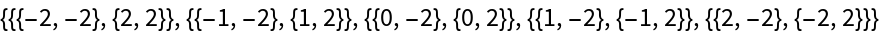Get the velocities over time:

 In:=Out=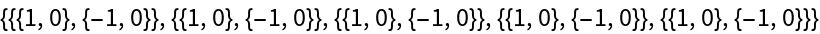Get the speeds over time:

 In:=Out=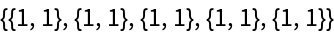Get the trajectories over time:

 In:=Out=Get the positions grouped by particle:

 In:=Out=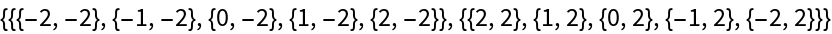Get the velocities grouped by particle:

 In:=Out=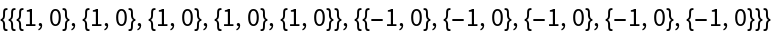Get the speeds grouped by particle:

 In:=Out=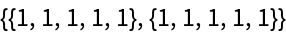Get the trajectories grouped by particle:

 In:=Out=### Scope (7)

Use trajectory data to visualize frames from a hard sphere simulation:

 In:=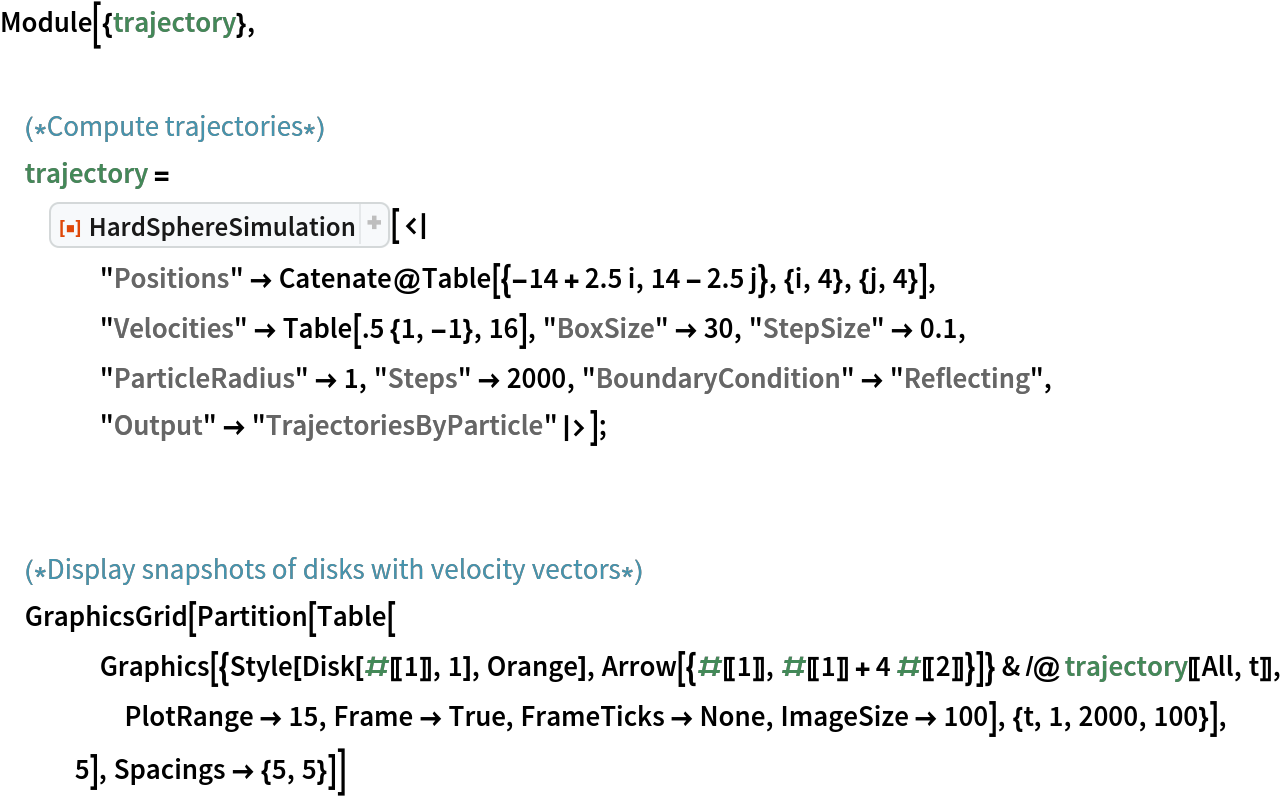Out=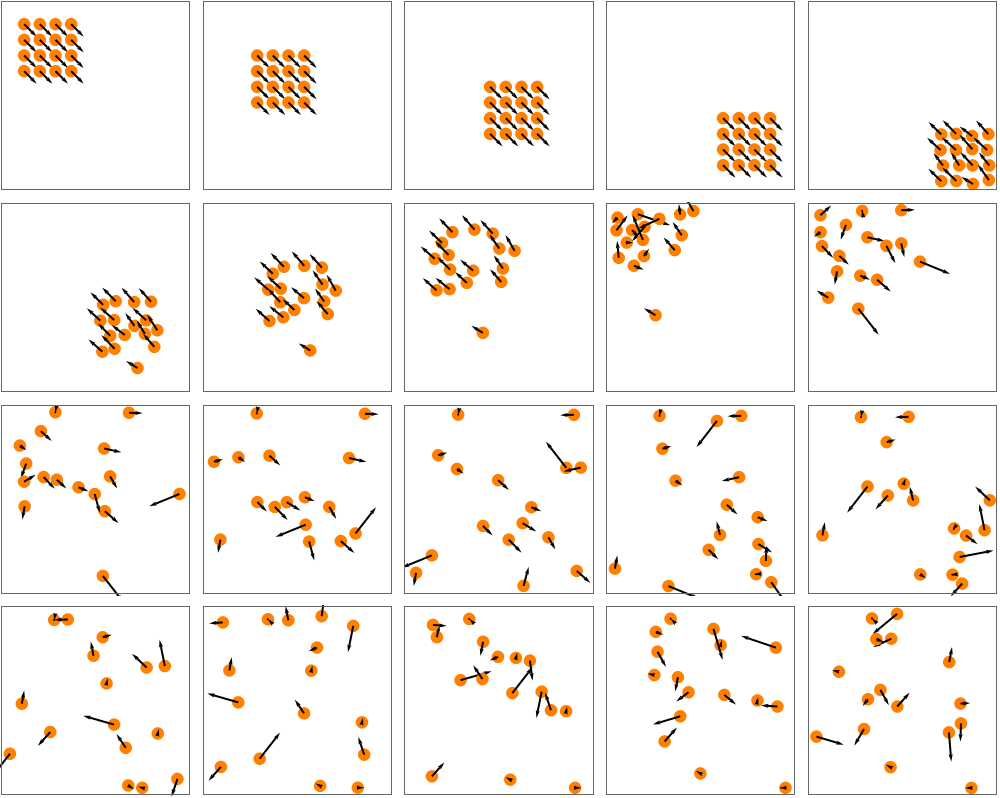Use "Visualize" to generate an interactive visualization of many hard spheres moving in a box:

 In:=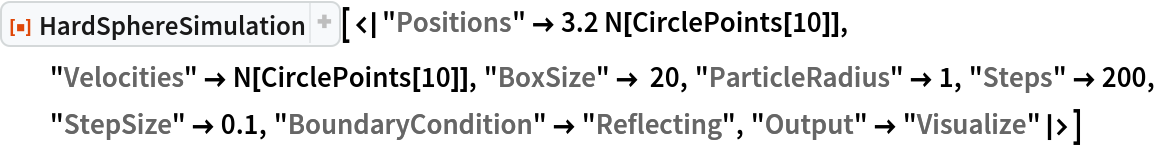Out=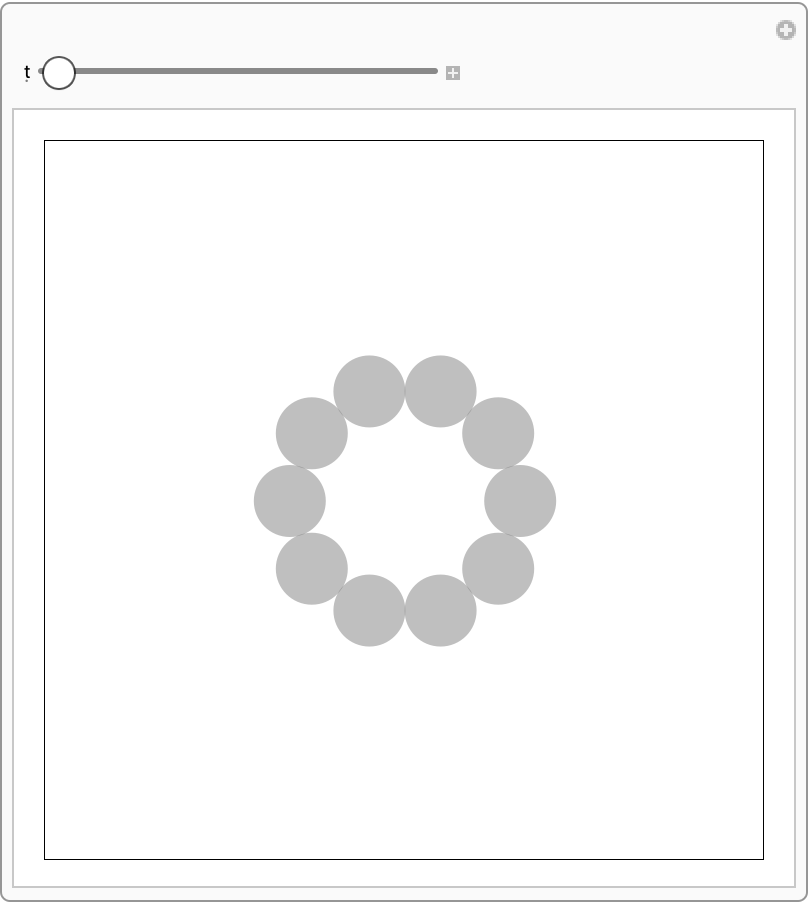Use "RandomNonOverlapping" to generate random non-overlapping initial positions in the box:

 In:=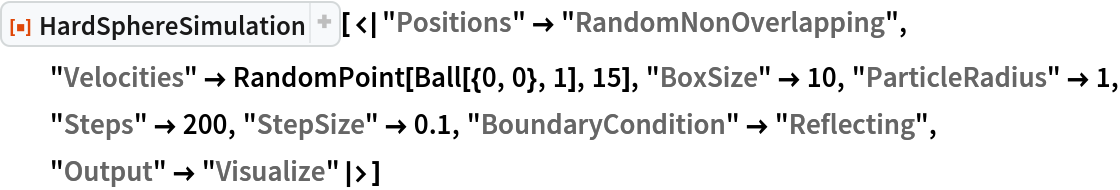Out=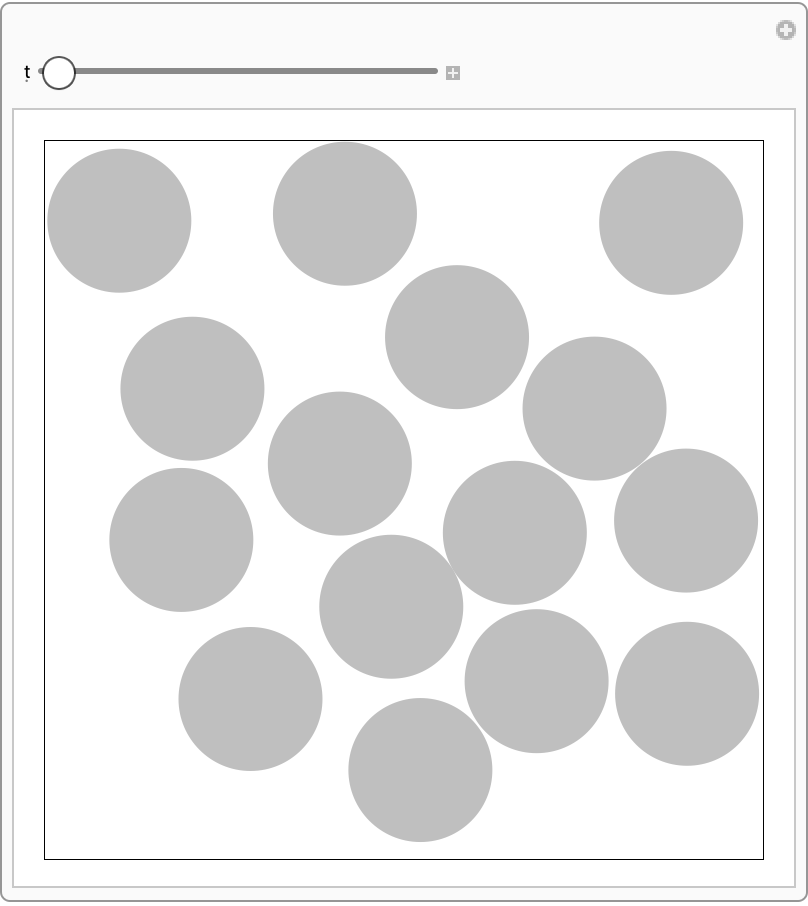Visualization works in 1D:

 In:=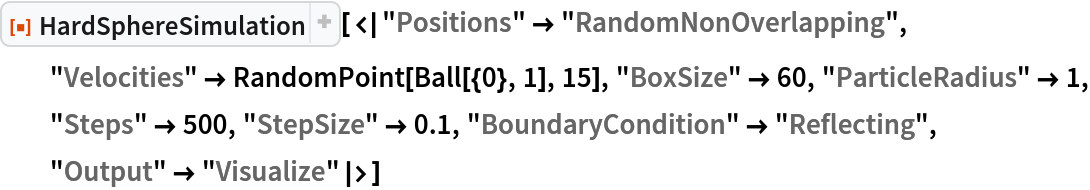Out=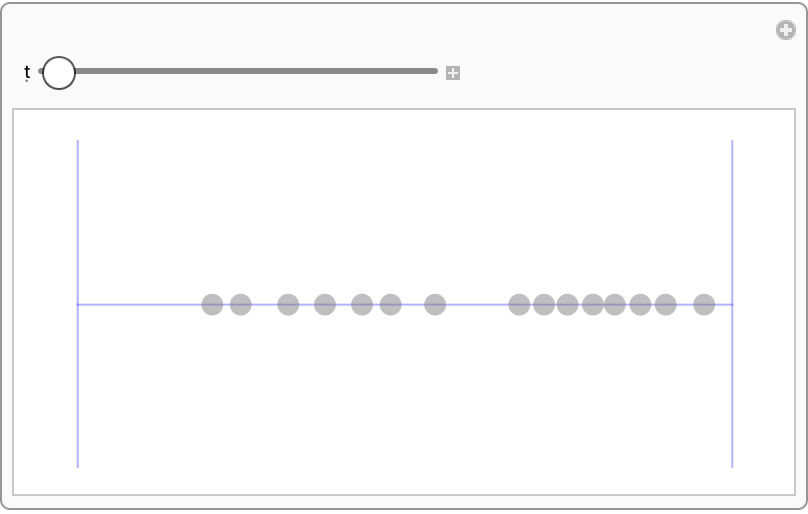Visualization works in 3D as well:

 In:=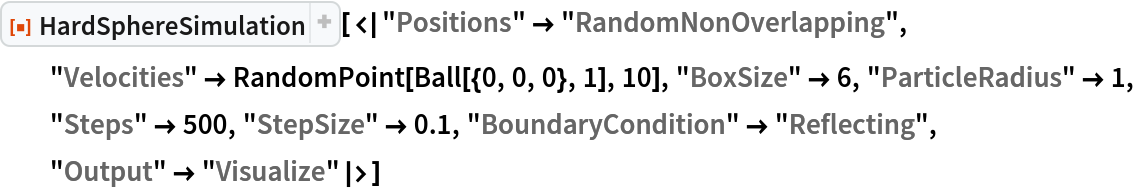Out=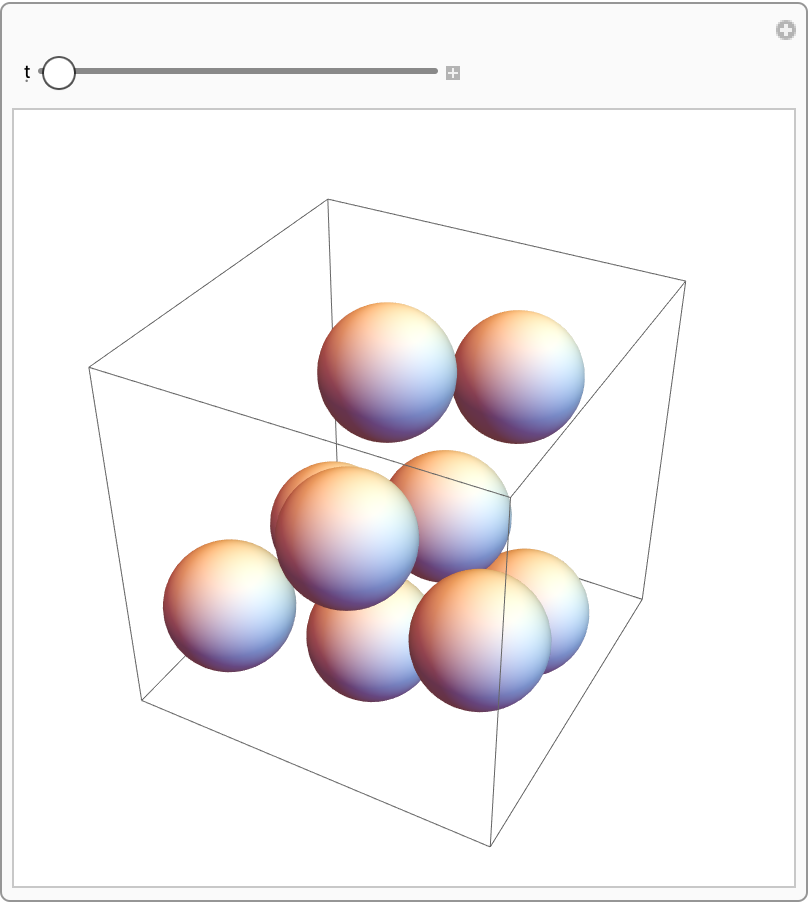Periodic boundary conditions identify opposite walls of the box with one another:

 In:=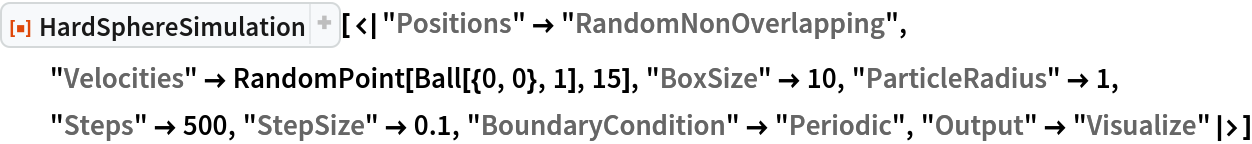Out=Infinite boundary conditions eliminate particle-wall interactions altogether:

 In:=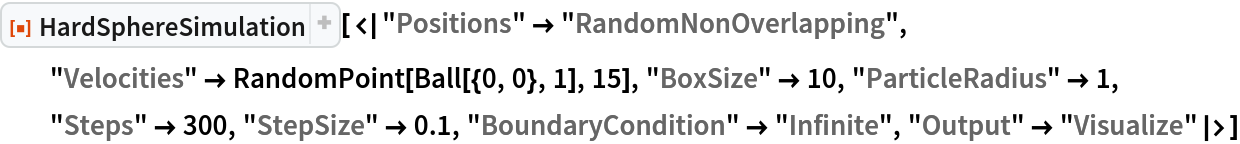Out=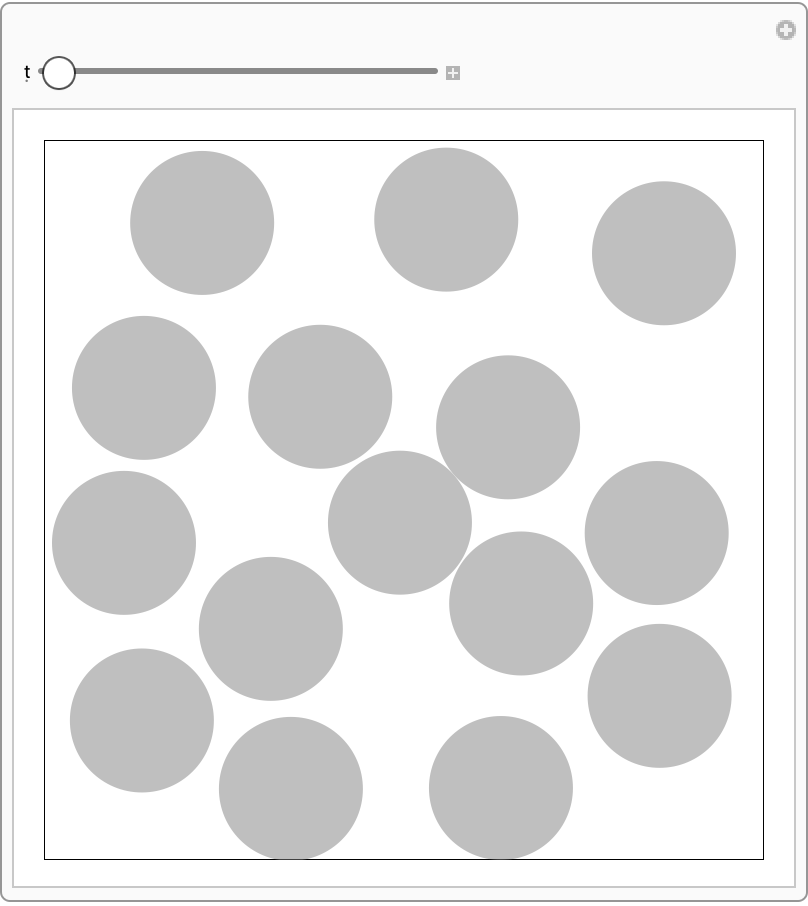Multiple outputs can be returned at once:

 In:=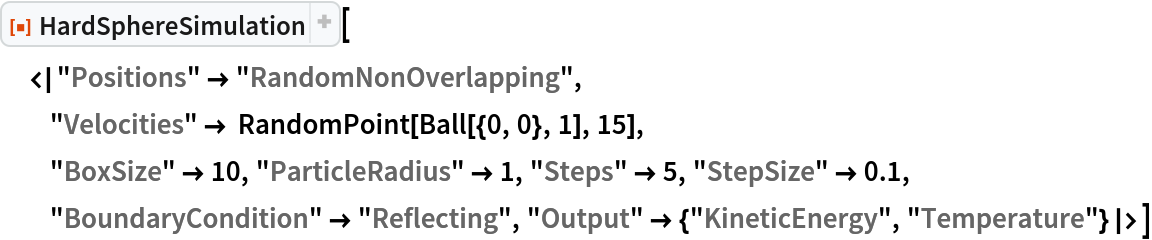Out=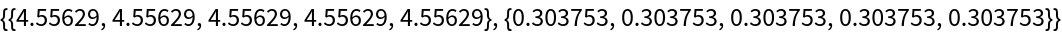### Applications (3)

Particle speeds should obey Maxwell-Boltzmann distribution:

 In:=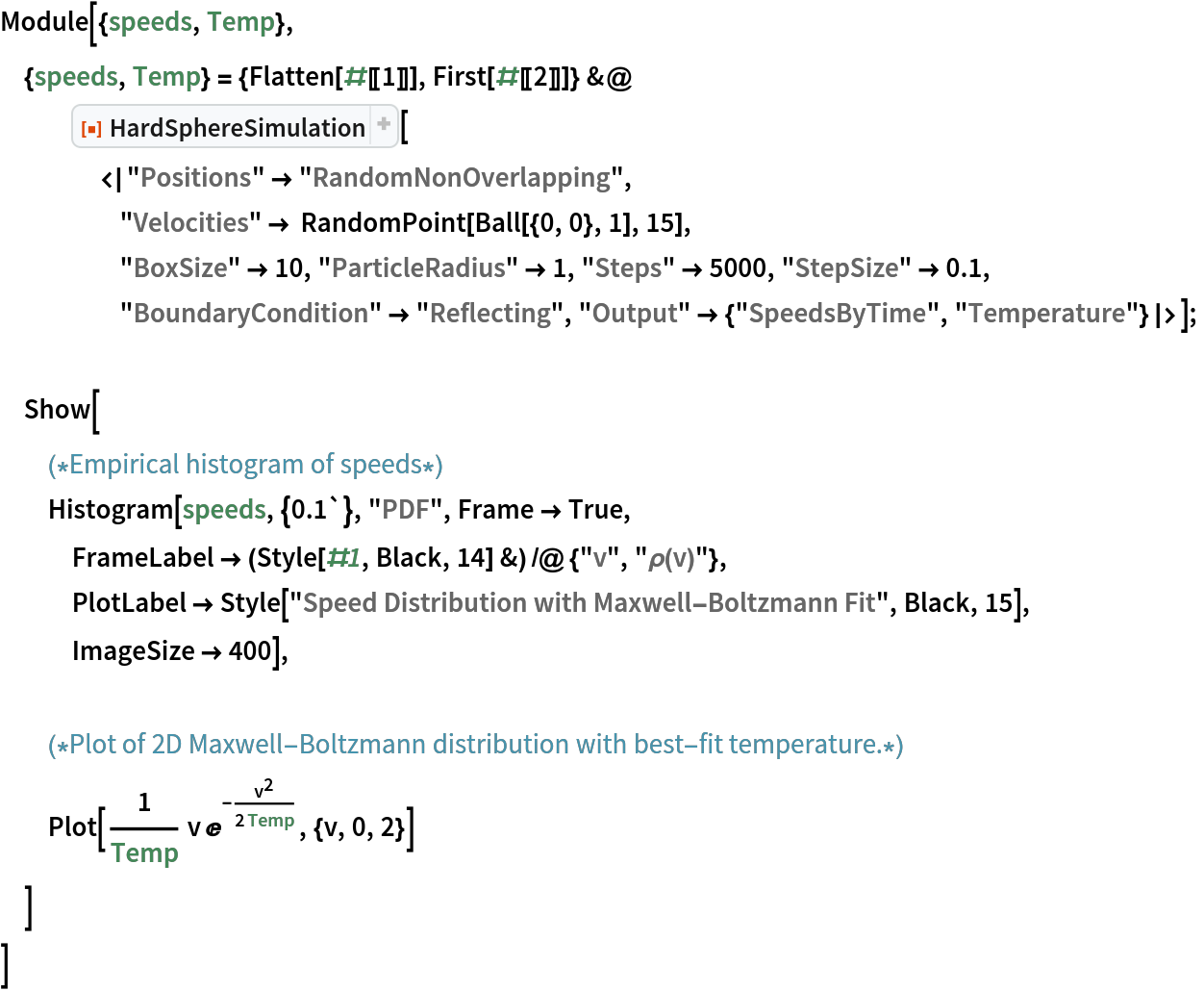Out=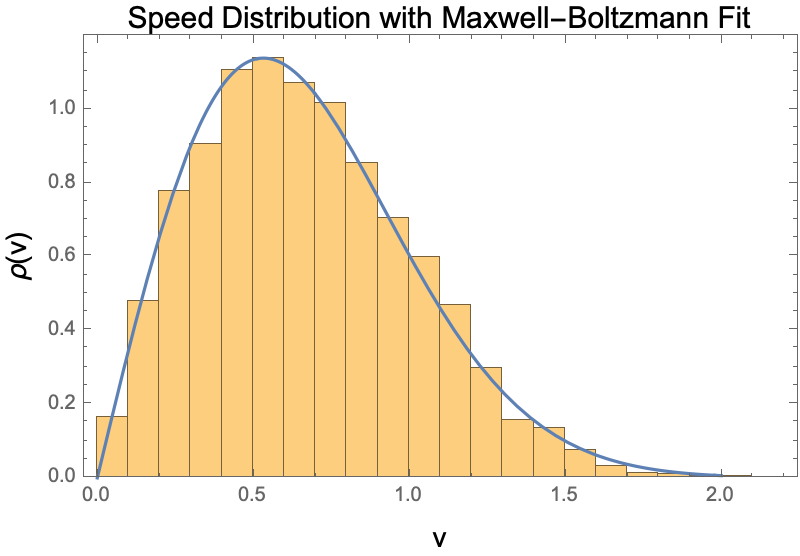Extract a collision list from the simulation, where collisions take the form {particle1,particle2,time of collision}:

 In:=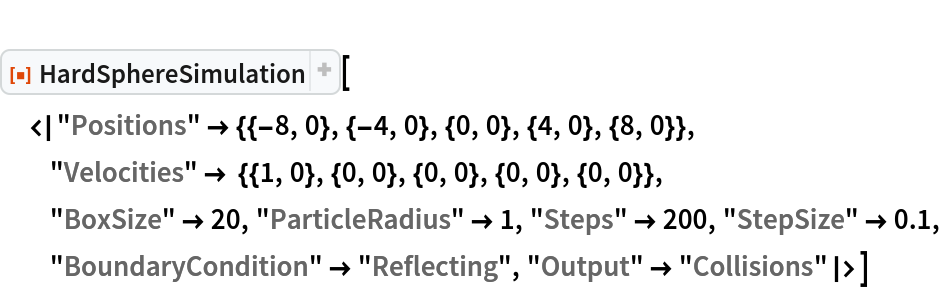Out=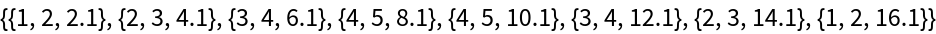From particle-particle collisions, construct the causal graph:

 In:=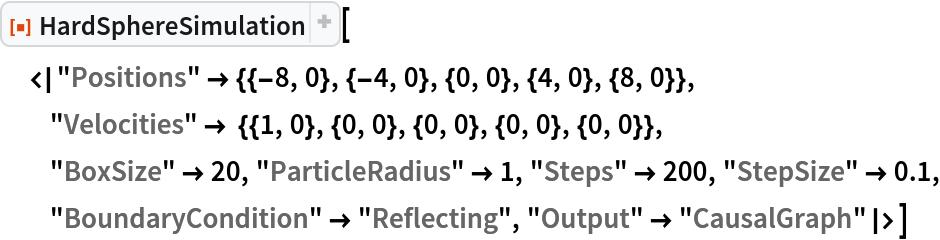Out=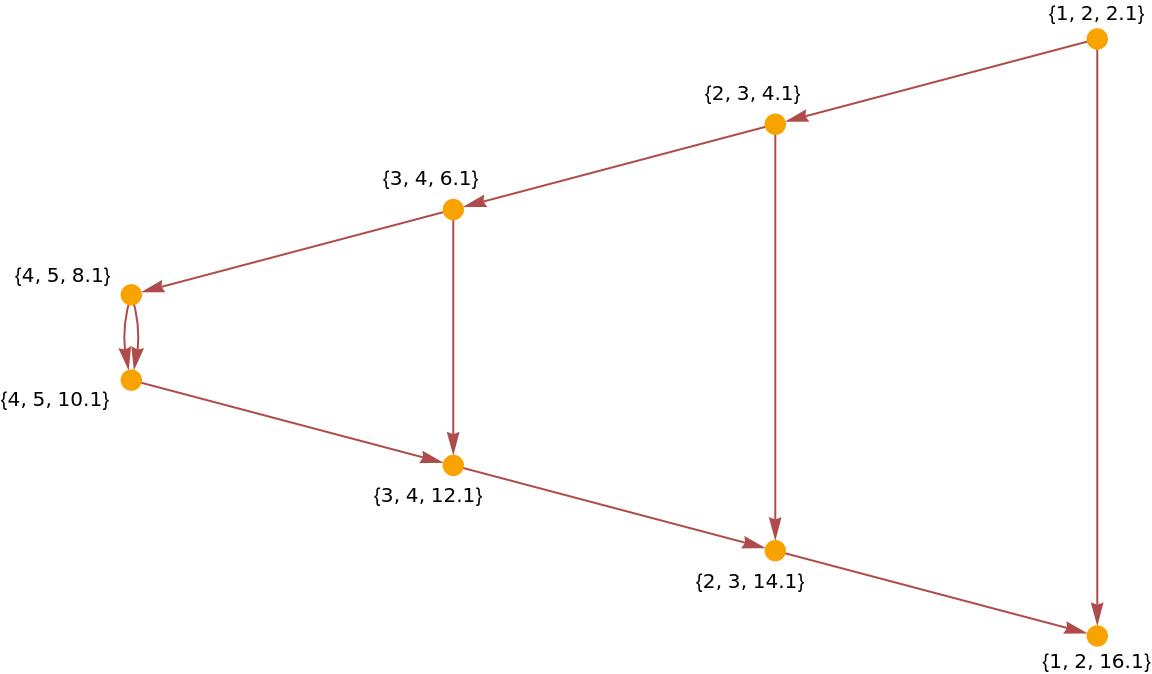Construct a causal graph for a disordered system:

 In:=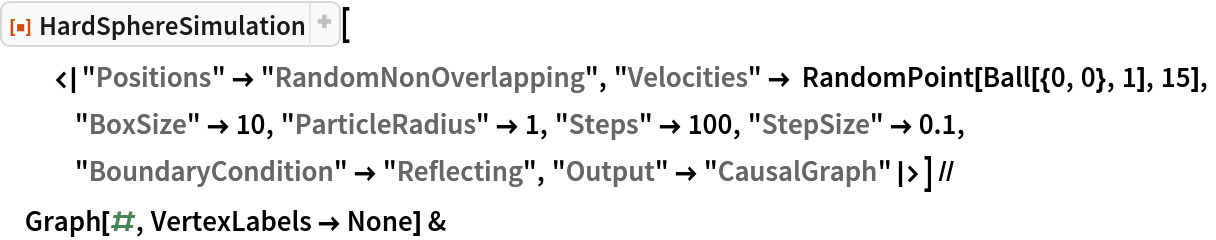Out=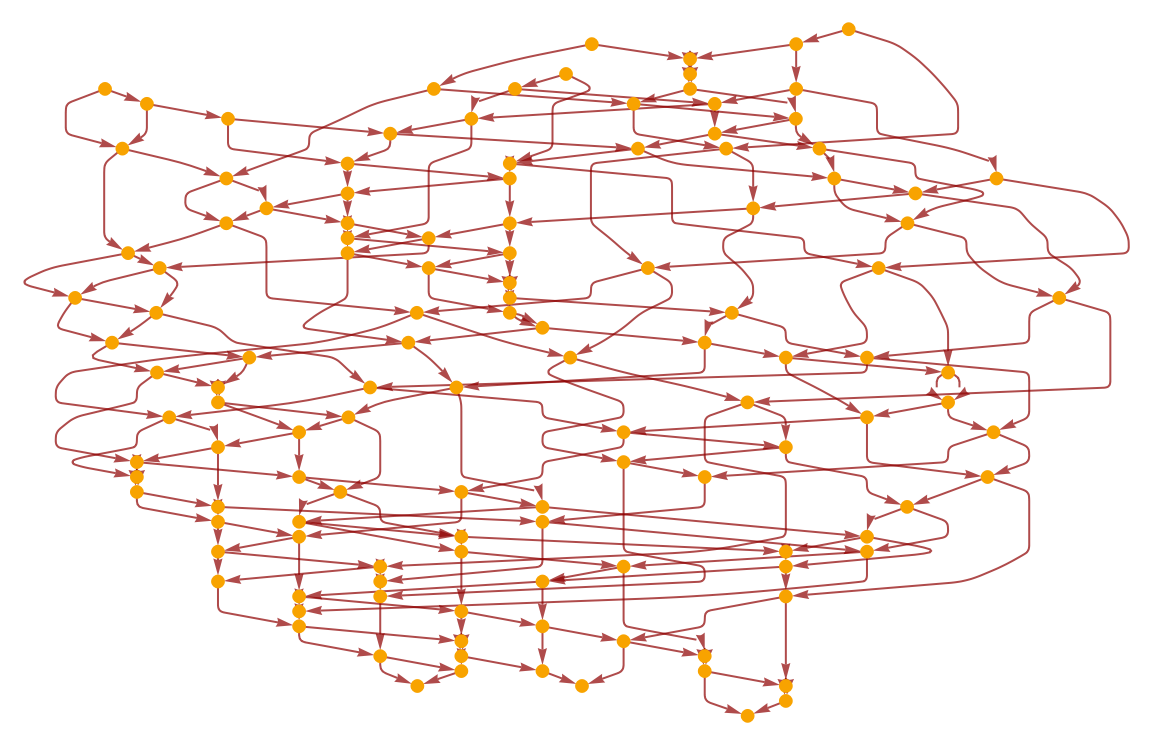As the nodes of the causal graph represent collisions between two particles and the edges represent individual particles, we expect all nodes to have indegree and outdegree 2, besides from the graph boundary (i.e., the beginning and end of the simulation):

 In:=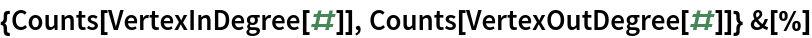Out=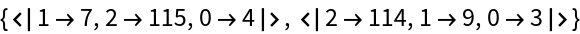### Properties and Relations (2)

Total kinetic energy of spheres should be conserved in time. Show that the error stays within machine precision:

 In:=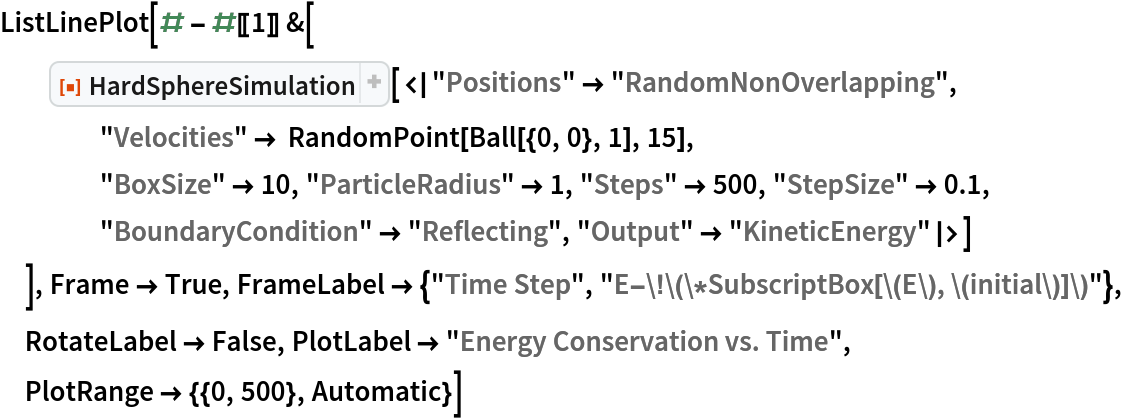Out=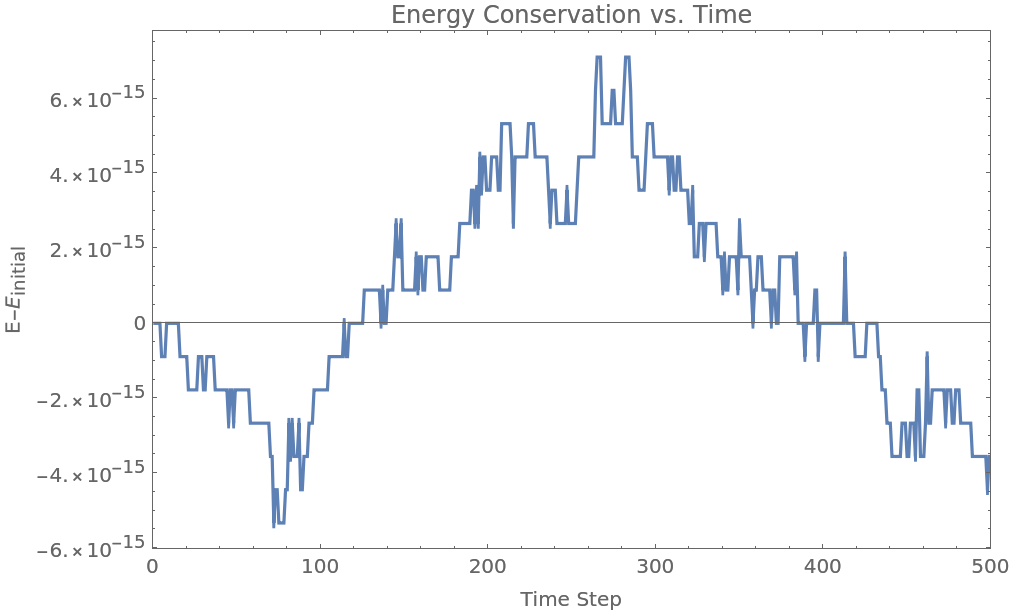Momentum should be conserved during collisions between particles. Collision of equal mass particles:

 In:=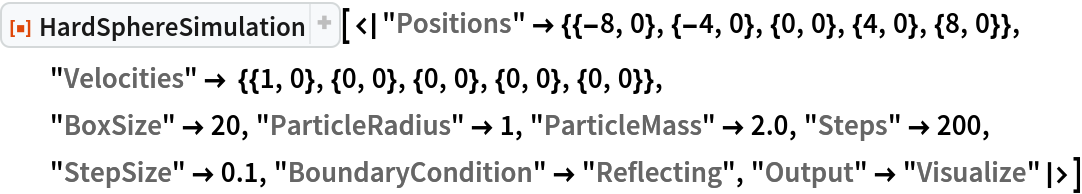Out=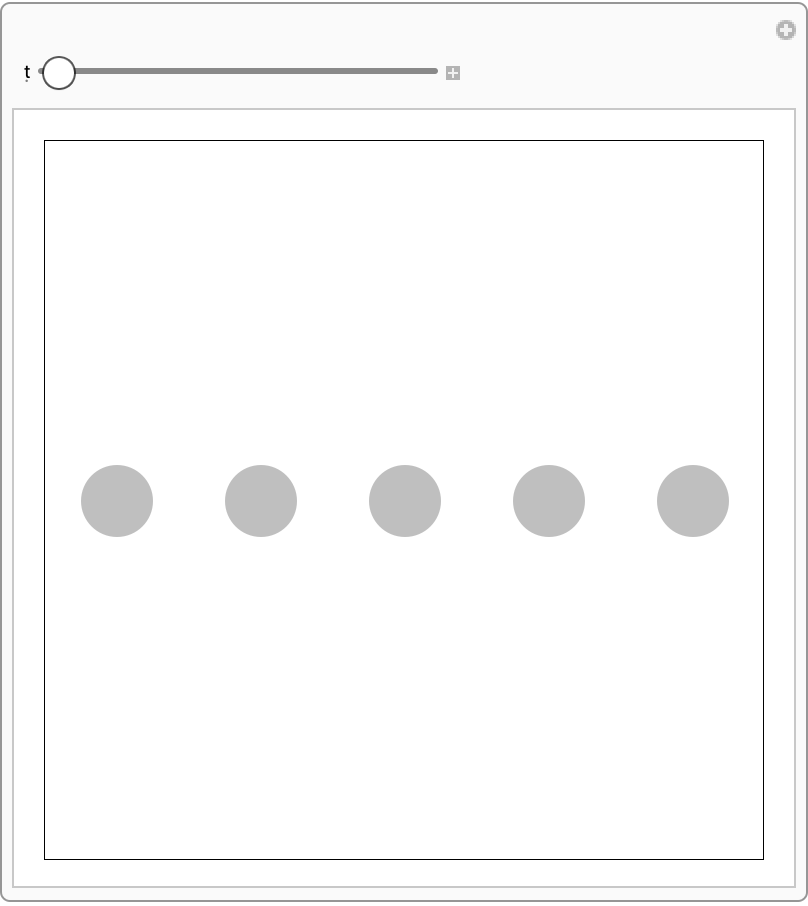Collision of an incoming heavy particle with a much lighter stationary particle causes the light particle to go out with double the speed of the incoming one:

 In:=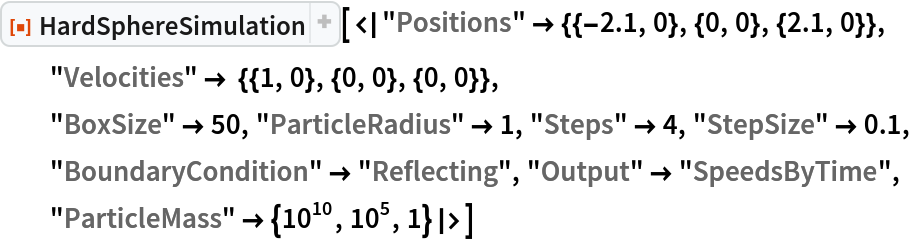Out=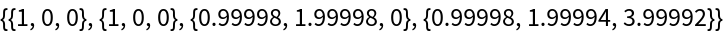Collision of a very light particle with a much heavier stationary particle leads to reflection of the incoming particle:

 In:=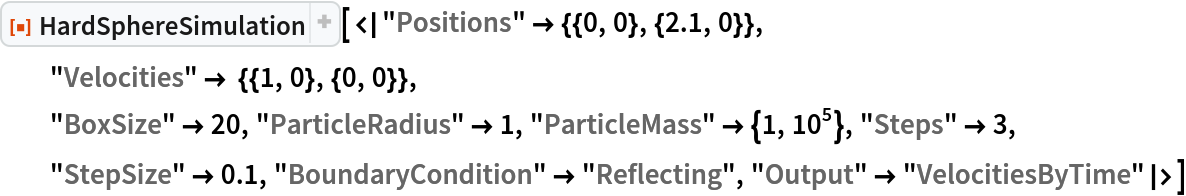Out=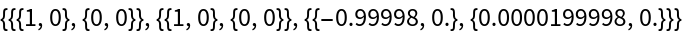### Possible Issues (2)

Particle overlaps are resolved pairwise, so situations involving multiple overlapping spheres may lead to unexpected results (such as a disruption of symmetry):

 In:=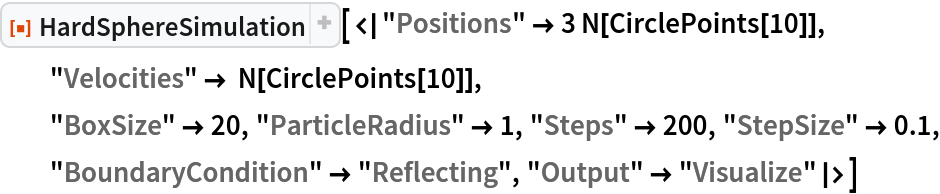Out=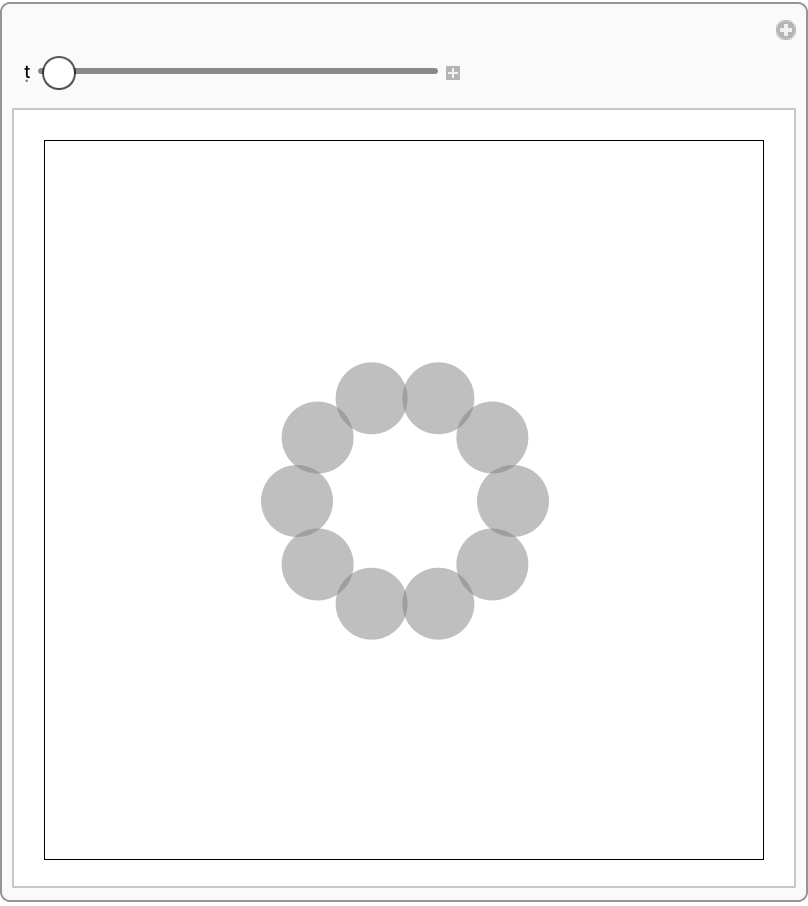The "RandomNonOverlapping" option is not guaranteed to find non-overlapping configurations at very high packing fractions:

 In:=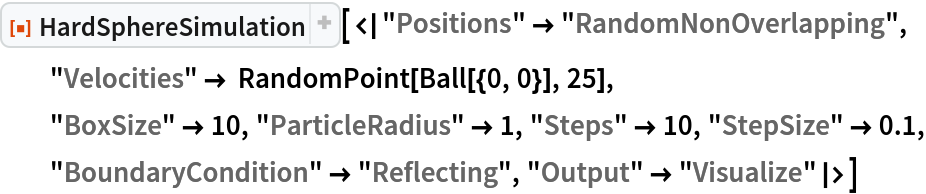Out=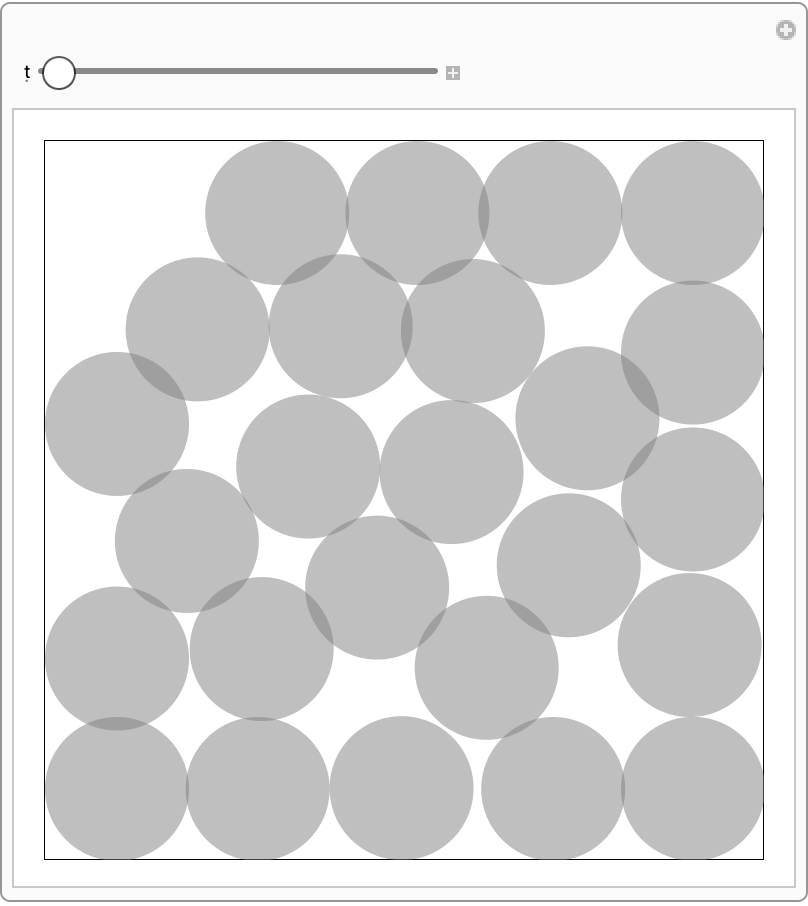### Neat Examples (2)

 In:=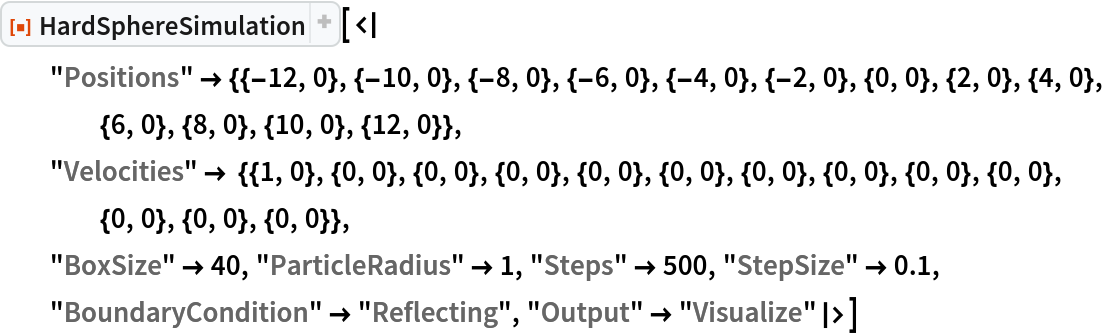Out=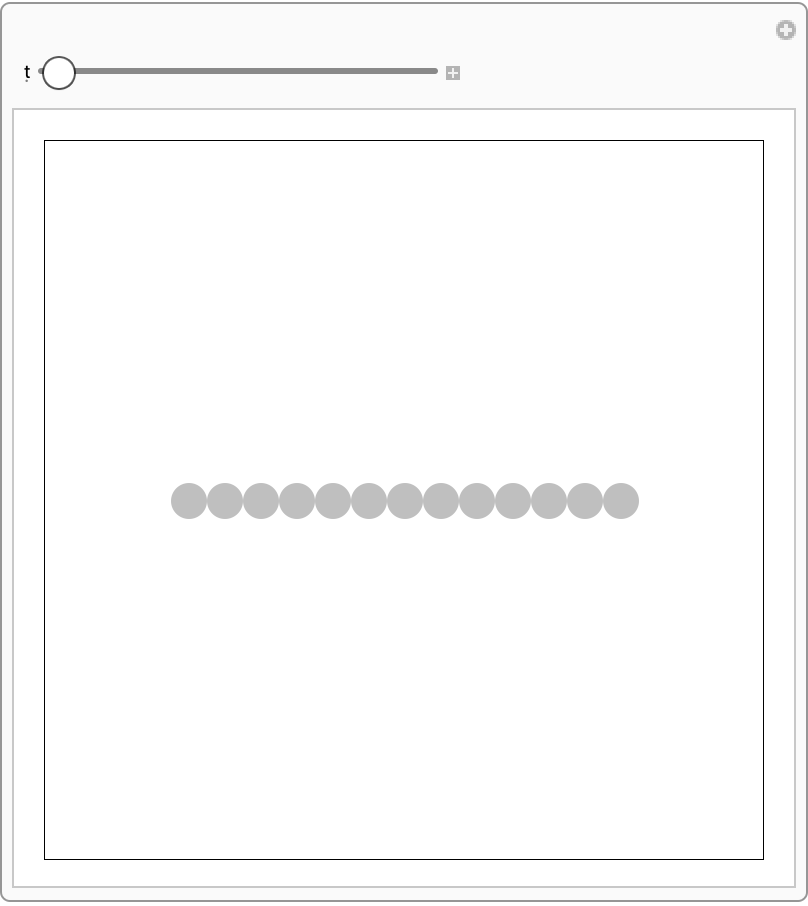Use HardSphereSimulation to simulate a simplified game of pool:

 In:=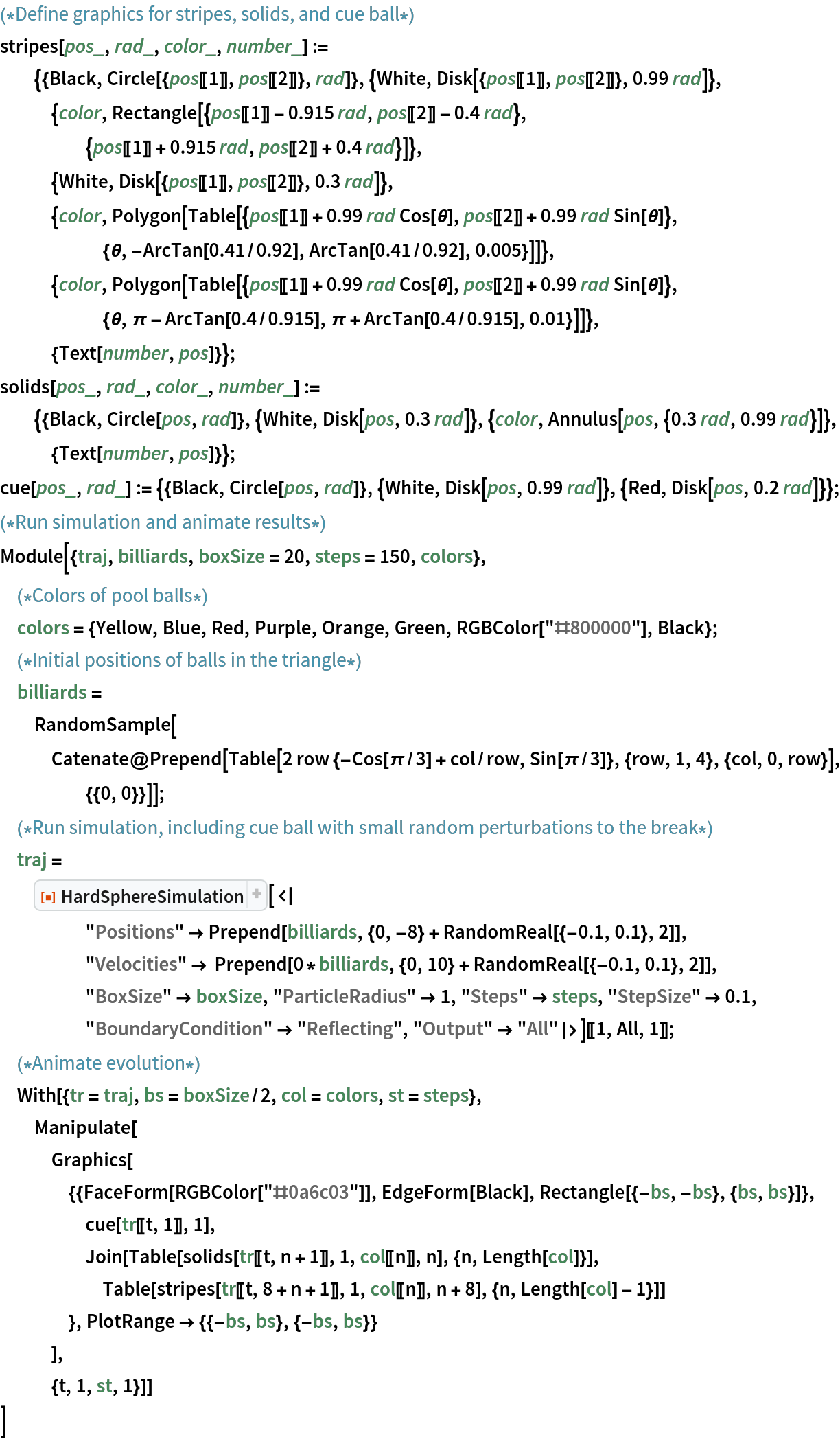Out=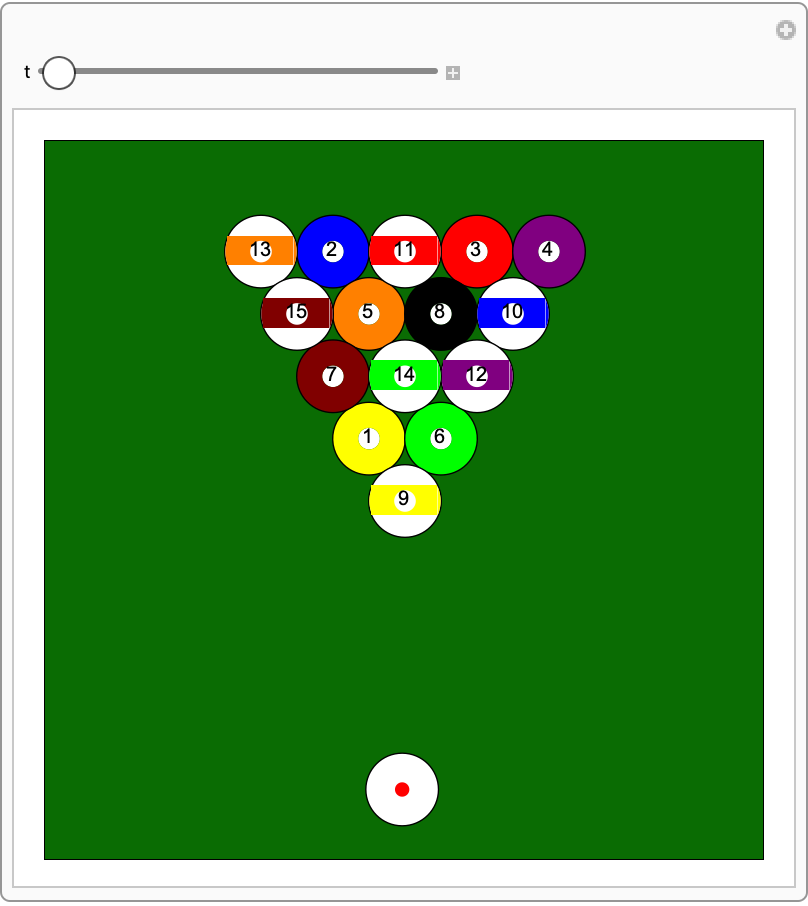## Publisher

Wolfram Summer School

## Version History

• 2.1.0 – 30 December 2022
• 2.0.1 – 14 October 2022
• 2.0.0 – 28 September 2022
• 1.0.0 – 16 February 2021